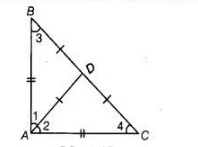# ABC is a right triangle with AB = AC.`
Question:

ABC is a right triangle with AB = AC. If bisector of ∠A meets BC at D,then prove that BC = 2AD.

Solution:

Given $\triangle A B C$ is a right angled triangle with $A B=A C, A D$ is the bisector of $\angle A$.To prove $B C=2 A D$ [given]

Proof $\ln \triangle A B C$,   $A B=A C$ [given]

$\Rightarrow$ $\angle C=\angle B$ $\ldots(1)$

[angles opposite to equal sides are equal]

Now, in right angled $\triangle A B C, \angle A+\angle B+\angle C=180^{\circ}$

[by angle sum property of a triangle in $180^{\circ}$ ]

$\Rightarrow \quad 90^{\circ}+\angle B+\angle B=180^{\circ}$ [from Eq. (i)]

$\Rightarrow \quad 2 \angle B=90^{\circ}$

$\Rightarrow \quad \angle B=45^{\circ}$

$\Rightarrow \quad \angle B=\angle C=45^{\circ}$

or $\quad \angle 3=\angle 4=45^{\circ}$

Now, $\angle 1=\angle 2=45^{\circ}$ $[\because A D$ is bisector of $\angle A]$

$\therefore \quad \angle 1=\angle 3 \angle 2=\angle 4$

$\Rightarrow \quad B D=A D, D C=A D$ ......(ii)

[sides opposite to equal angles are equal]

Hence, $B C=B D+C D=A D+A D \Rightarrow B C=2 A D$ [from Eq. (ii)]

Hence proved.